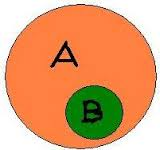# Number Of Elements In A Subset

Probability Level 3A subsets $B$ of the set $A=\{1,2,3,\ldots, 100\}$ has the property that no pair of distinct numbers in $B$ has a sum divisible by 7.

What is the largest number of elements that $B$ could have?

×